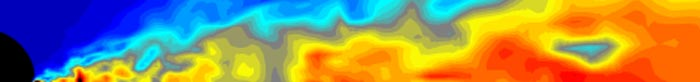Fall 2009: Special Relativity and Waves (171.201/171.207)

Overview: Special Relativity and Waves is the third course in the four-semester introductory sequence for physics majors. The course is divided into two parts. In the first three-four weeks we study the theory of special relativity (this is where 171.207 course ends after the first midterm and relevant homework). Then the rest of the semester is devoted to the physics of waves (for those who take the full course 171.201). The course builds upon the background in classical mechanics and electromagnetism, and precedes the full development of quantum physics.

Required texts:

• "Introduction to Special Relativity"
Author: Robert Resnick
Published: John Wiley and Sons
• "The Physics of Vibrations and Waves," 6th edition
Author: H. J. Pain
Published: John Wiley and Sons

Syllabus
Schedule

Part I: Special Relativity

Lecture 1 (Sep. 2, 2009): Introduction: course overview, schedule and syllabus.
Introduction to Special Relativity: Galilean transformations, Maxwell equations, problems with relativity in EM. Michelson-Morley experiment.
Demo: Michelson interferometer (for both special relativity and waves)
Chapter 1 of Resnick

Lecture 2 (Sep. 4, 2009): Postulates of Special Relativity. Lorentz transformations. Length contraction and time dilation.
Chapters 2.1-2.3 of Resnick

Lecture 3 (Sep. 9, 2009): Lorentz transformations. More examples. Minkowski space-time.
Demo: Movie
Chapters 2.4-2.5, A.1-A.2 of Resnick

Lecture 4 (Sep. 11, 2009): More on Minkowski space-time. Twin paradox and "time travel."
Chapters A.3, B of Resnick

Lecture 5 (Sep. 14, 2009): Addition of Velocities. Doppler effect and Aberration.
Chapters 2.6-2.8 of Resnick

Lecture 6 (Sep. 16, 2009): Relativistic dynamics. Equivalence of mass and energy, relativistic mass.
Chapters 3.1-3.5 of Resnick

Lecture 7 (Sep. 18, 2009): Charged particle in electric and magnetic fields. Transformation of momentum, energy, and mass between frames.
Chapters 3.5, 3.7 of Resnick

Lecture 8 (Sep. 21, 2009): More examples of relativistic dynamics. EM theory and relativity.
Chapters 3.6-3.7, 4.1-4.2 of Resnick

Lecture 9 (Sep. 23, 2009): Transformation of force. EM field transformation and field of a moving charge.
Chapters 4.3-4.4 of Resnick

Lecture 10 (Sep. 25, 2009): Photon and EM effects. Equivalence principle of General Relativity.
Chapters 4, C (selected topics) of Resnick

Part II: Waves

Lecture 11 (Sep. 28, 2009): Intro to Waves. Simple harmonic oscillations. Complex numbers. Energy of a harmonic oscillator.
Demos
Chapter 1 of Pain

Lecture 12 (Sep. 30, 2009): Damped harmonic oscillations.
Demos
Chapter 2 of Pain

Lecture 13 (Oct. 2, 2009): Quality factor of damped harmonic oscillations. Superposition of waves.
Demos
Chapter 2 of Pain

Lecture 14 (Oct. 5, 2009): Electric circuits. Forced oscillations.
Demos
Chapter 3 of Pain

Lecture 15 (Oct. 7, 2009): More on forced oscillations.
Demo
Chapter 3 of Pain

Lecture 16 (Oct. 9, 2009): MIDTERM EXAM on Special Relativity

Lecture 17 (Oct. 12, 2009): Coupled oscillations.
Demos
Chapter 4 of Pain

Lecture 18 (Oct. 14, 2009): More on coupled oscillations, forced coupled oscillations, many-body coupled oscillations.
Demos
Chapter 4 of Pain

Lecture 19 (Oct. 16, 2009): Many-body coupled oscillations and wave equation.
Demos
Chapters 4 and beginning of 5 of Pain

Lecture 20 (Oct. 19, 2009): Waves. Progressive waves. Standing waves.
Demos
Chapter 5 of Pain

Lecture 21 (Oct. 21, 2009): Impedance of a string. Transmission and reflection of waves.
Demos
Chapter 5 of Pain

Lecture 22 (Oct. 23, 2009): Matching impedance. Group and phase velocity.
Demo (computer simulation)
Chapter 5 of Pain

Lecture 23 (Oct. 26, 2009): More on group and phase velocity. Energy in a normal mode of a standing wave. Idea of Fourier series.
Chaptera 5 and beginning of 10 of Pain

Lecture 24 (Oct. 28, 2009): Fourier series. Application to standing waves.
Demos
Chapter 10 of Pain

Lecture 25 (Oct. 30, 2009): Fourier transformations.
Demos
Chapter 10 of Pain

Lecture 26 (Nov. 2, 2009): Applications of Fourier transformations.
Chapters 10 and 5 of Pain

Lecture 27 (Nov. 4, 2009): More on Fourier transformations. The uncertainty principle. Sound waves in gases.
Chapters 10 and 5, touch on Chapters 13, Move to Chapter 6 of Pain

Lecture 28 (Nov. 6, 2009): More on sound waves.
Demos
Chapter 6 of Pain

Lecture 29 (Nov. 9, 2009): More on sound waves. Transmission lines.
Demos
Chapters 6, 7 of Pain

Lecture 30 (Nov. 11, 2009): More on transmission lines. Resistance in transmission lines. Waves in 2D.
Demos
Chapter 7 of Pain

Lecture 31 (Nov. 13, 2009): MIDTERM EXAM on Waves

Lecture 32 (Nov. 16, 2009): Waves in 2D and 3D. Waveguides. Separation of variables. Standing waves in 2D.
Demos
Chapter 9 of Pain

Lecture 33 (Nov. 18, 2009): Electromagnetic waves: spectrum, wave equation from Maxwell equations, polarized light.
Demos
Chapter 8 of Pain

Lecture 34 (Nov. 20, 2009): Electromagnetic waves: energy in a wave, reflection and transmission at a boundary, Snell's law
Chapter 8 of Pain

Lecture 35 (Nov. 23, 2009): Electromagnetic waves: reflection and transmission at an angle, Brewster angle and total internal reflection, comment on impedance of a conductor, interference.
Chapter 8, 12 of Pain

Lecture 36 (Nov. 30, 2009): EM waves: interference with division of amplitude in a glass, division of wavefront, interference from two sources, limit on coherence.
Demos
Chapter 12 of Pain

Lecture 37 (Dec. 2, 2009): EM waves: interference from N sources, diffraction, interference with diffraction fron N slits.
Demo: Michelson interferometer
Chapter 12 of Pain

Lecture 38 (Dec. 4, 2009): Intro to wave mechanics
Chapter 13 of Pain

Andrei Gritsan
Last update: Thu Dec 17 14:24:38 EST 2009